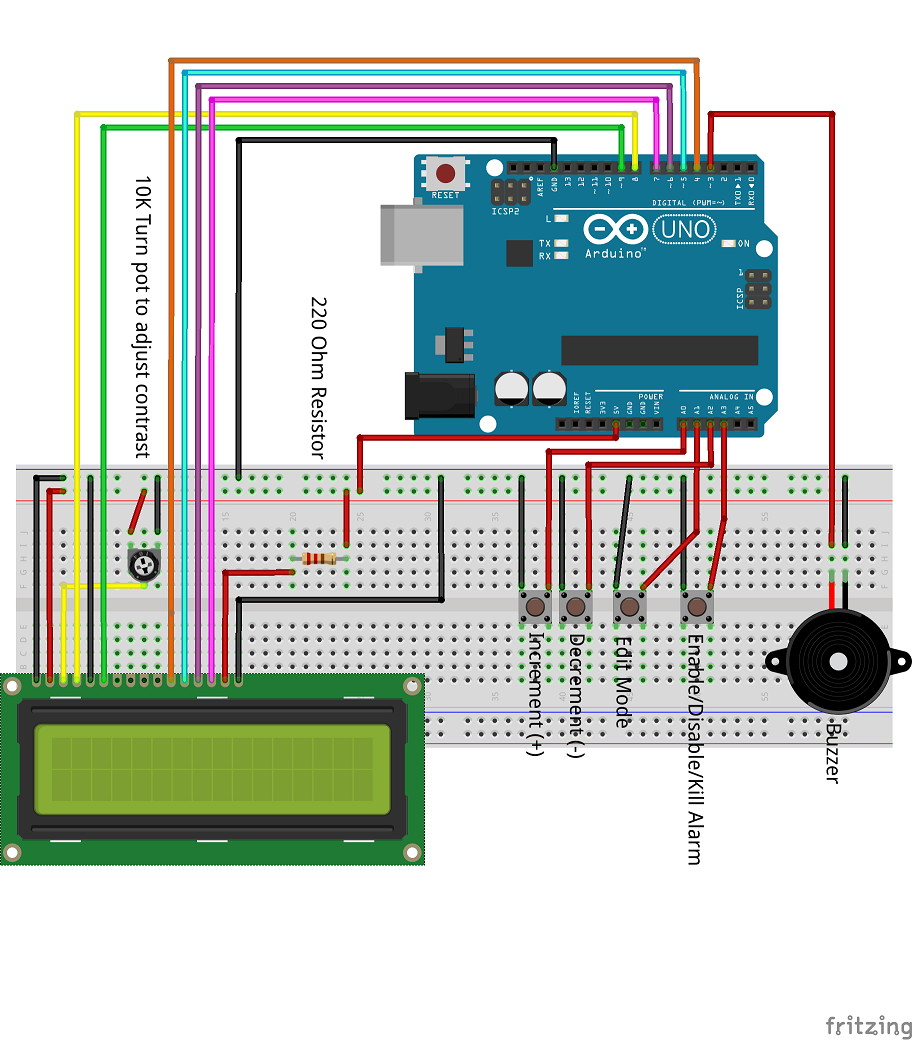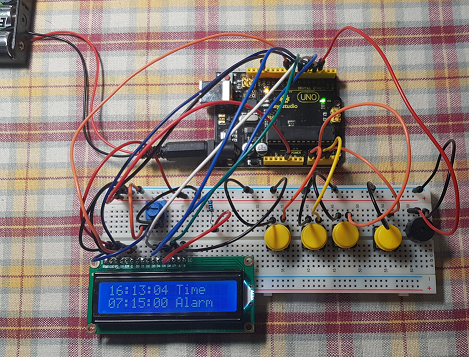B4R TutorialDigital Clock and Alarm without using a RTC

A digital clock and alarm without making use of a RTC. It uses:
Uno R3
LCD 1602 (to display the clock and the alarm)
Piezo Buzzer (to sound the preset alarm)
10K Pot (adjust the contrast of the LCD display)
220 Ohm resistor (make it bigger to dim the backlight intensity more)
4 x buttons (from left to right in the below image - increment value, decrement value, edit mode, alarm on/off)
Numerous wires

It uses libraries:
rCore
rLiquidCrystal

The bell custom character is created and displayed via inline C code

Wiring diagram:Edit Alarm Minutes - alarm is activeClock running and alarm set, and alarm activated (bell showing)Clock running, alarm set, but alarm not activated (bell not showing)Press the edit mode button (3rd from left) to edit hours, minutes, and seconds of the time or of the alarm (every edit/mode button press will take you to the next value to be adjusted)
Use the increment (first from left) and decrement (second from left) buttons to adjust/set hours, minutes, seconds of the time and the alarm when edit mode has been activated.
Use the right most button to activate/deactivate the alarm. This button will also silence the alarm when the alarm is ringing.

Sample Code:

B4X:
#Region Project Attributes
#AutoFlushLogs: True
#StackBufferSize: 300
#End Region

Sub Process_Globals
Public Serial1 As Serial
Private hours, minutes, seconds As Int = 0
Dim alarmhours, alarmminutes, alarmseconds As Int = 0
Private timer1 As Timer
Private lcd As LiquidCrystal
Dim pa0, pa1, pa2, pa3, buzzerpin As Pin
Dim hour_changed, minute_changed, second_changed As Boolean = False
Dim alarmhour_changed, alarmminute_changed, alarmsecond_changed As Boolean = False
Dim mode_changed As Boolean = False
Dim mode As Int = 0
Dim alarm_active As Boolean = False

End Sub

Private Sub AppStart
Serial1.Initialize(115200)
Log("AppStart")
timer1.Initialize("Timer1_Tick", 1000)

pa0.Initialize(pa0.A0, pa0.MODE_INPUT_PULLUP)           'increment time depending on edit mode
pa1.Initialize(pa1.A1, pa1.MODE_INPUT_PULLUP)           'select edit mode
pa2.Initialize(pa2.A2, pa1.MODE_INPUT_PULLUP)           'decrement time depending on edit mode
pa3.Initialize(pa3.A3, pa3.MODE_INPUT_PULLUP)
buzzerpin.Initialize(3, buzzerpin.MODE_OUTPUT)
buzzerpin.DigitalWrite(False)

lcd.Initialize(8, 255, 9, Array As Byte (4, 5, 6, 7))
lcd.Begin(16, 2)
timer1.Enabled = True

'set time and alarm initial values
hours = 16
minutes = 48
seconds = 0
alarmhours = 07
alarmminutes = 15
alarmseconds = 0

lcd.SetCursor(9,0)
lcd.Write("Time")
lcd.SetCursor(9,1)
lcd.Write("Alarm")
RunNative("createCharsAlarm", Null)        'create an alarm / bell character

End Sub

Sub pa3_StateChanged

Delay(20)

If mode = 0 Then
If alarm_active = False Then
alarm_active = True
lcd.SetCursor(15,1)
RunNative("writeCharAlarm", 0)   'display the Bell special character
End If
Else
alarm_active = False
buzzerpin.DigitalWrite(False)
lcd.SetCursor(15,1)
lcd.Write(" ")
End If
End If
End If

End Sub

Sub pa0_StateChanged
Delay(20)

If mode = 1 Then                             'edit mode 1 - set the hours of the time
If Not(hour_changed) Then
hours = hours + 1
If hours = 24 Then hours = 0
lcd.Write(NumberFormat(hours, 2, 0))
lcd.SetCursor(0,0)
hour_changed = Not(hour_changed)
Else
hour_changed = Not(hour_changed)
End If
End If

If mode = 2 Then
If Not(minute_changed) Then
minutes = minutes + 1
If minutes = 60 Then minutes = 0
lcd.Write(NumberFormat(minutes, 2, 0))
lcd.SetCursor(3,0)
minute_changed = Not(minute_changed)
Else
minute_changed = Not(minute_changed)
End If
End If

If mode = 3 Then
If Not(second_changed) Then
seconds = seconds + 1
If seconds = 60 Then seconds = 0
lcd.Write(NumberFormat(seconds, 2, 0))
lcd.SetCursor(6,0)
second_changed = Not(second_changed)
Else
second_changed = Not(second_changed)
End If
End If

If mode = 4 Then
If Not(alarmhour_changed) Then
alarmhours = alarmhours + 1
If alarmhours = 24 Then alarmhours = 0
lcd.Write(NumberFormat(alarmhours, 2, 0))
lcd.SetCursor(0,1)
alarmhour_changed = Not(alarmhour_changed)
Else
alarmhour_changed = Not(alarmhour_changed)
End If
End If

If mode = 5 Then
If Not(alarmminute_changed) Then
alarmminutes = alarmminutes + 1
If alarmminutes = 60 Then alarmminutes = 0
lcd.Write(NumberFormat(alarmminutes, 2, 0))
lcd.SetCursor(3,1)
alarmminute_changed = Not(alarmminute_changed)
Else
alarmminute_changed = Not(alarmminute_changed)
End If
End If

If mode = 6 Then
If Not(alarmsecond_changed) Then
alarmseconds = alarmseconds + 1
If alarmseconds = 60 Then alarmseconds = 0
lcd.Write(NumberFormat(alarmseconds, 2, 0))
lcd.SetCursor(6,1)
alarmsecond_changed = Not(alarmsecond_changed)
Else
alarmsecond_changed = Not(alarmsecond_changed)
End If
End If

End Sub

Sub pa2_StateChanged
Delay(20)

If mode = 1 Then
If Not(hour_changed) Then
hours = hours - 1
If hours = -1 Then hours = 23
lcd.Write(NumberFormat(hours, 2, 0))
lcd.SetCursor(0,0)
hour_changed = Not(hour_changed)
Else
hour_changed = Not(hour_changed)
End If
End If

If mode = 2 Then
If Not(minute_changed) Then
minutes = minutes - 1
If minutes = -1 Then minutes = 59
lcd.Write(NumberFormat(minutes, 2, 0))
lcd.SetCursor(3,0)
minute_changed = Not(minute_changed)
Else
minute_changed = Not(minute_changed)
End If
End If

If mode = 3 Then
If Not(second_changed) Then
seconds = seconds - 1
If seconds = -1 Then seconds = 59
lcd.Write(NumberFormat(seconds, 2, 0))
lcd.SetCursor(6,0)
second_changed = Not(second_changed)
Else
second_changed = Not(second_changed)
End If
End If

If mode = 4 Then
If Not(alarmhour_changed) Then
alarmhours = alarmhours - 1
If alarmhours = -1 Then alarmhours = 23
lcd.Write(NumberFormat(alarmhours, 2, 0))
lcd.SetCursor(0,1)
alarmhour_changed = Not(alarmhour_changed)
Else
alarmhour_changed = Not(alarmhour_changed)
End If
End If

If mode = 5 Then
If Not(alarmminute_changed) Then
alarmminutes = alarmminutes - 1
If alarmminutes = -1 Then alarmminutes = 59
lcd.Write(NumberFormat(alarmminutes, 2, 0))
lcd.SetCursor(3,1)
alarmminute_changed = Not(alarmminute_changed)
Else
alarmminute_changed = Not(alarmminute_changed)
End If
End If

If mode = 6 Then
If Not(alarmsecond_changed) Then
alarmseconds = alarmseconds - 1
If alarmseconds = -1 Then alarmseconds = 59
lcd.Write(NumberFormat(alarmseconds, 2, 0))
lcd.SetCursor(6,1)
alarmsecond_changed = Not(alarmsecond_changed)
Else
alarmsecond_changed = Not(alarmsecond_changed)
End If
End If

End Sub

Sub pa1_StateChanged
Delay(20)

timer1.Enabled = False                            'disable the timer while we adjuts the time or alamr
If Not(mode_changed) Then
mode_changed = Not(mode_changed)
mode = mode + 1                               'increase the mode (by default the mode = 0)
Else
mode_changed = Not(mode_changed)
End If

If mode = 1 Then                                  'mode to edit hours of time
lcd.SetCursor(0,0)
lcd.CursorOn = True
else if mode = 2 Then
lcd.SetCursor(3,0)
lcd.CursorOn = True
else if mode = 3 Then
lcd.SetCursor(6,0)
lcd.CursorOn = True
else if mode = 4 Then                            'mode to edit hours of alarm
lcd.SetCursor(0,1)
lcd.CursorOn = True
else if mode = 5 Then
lcd.SetCursor(3,1)
lcd.CursorOn = True
else if mode = 6 Then
lcd.SetCursor(6,1)
lcd.CursorOn = True
Else
mode = 0
lcd.CursorOn = False
timer1.Enabled = True
End If

End Sub

Sub Timer1_Tick

seconds = seconds + 1

If seconds = 60 Then
seconds = 0
minutes = minutes + 1
End If

If minutes = 60 Then
minutes = 0
hours = hours + 1
End If
If hours = 24 Then
hours = 0
End If

lcd.SetCursor(0,0)
lcd.Write(NumberFormat(hours, 2, 0))
lcd.Write(":")
lcd.Write(NumberFormat(minutes, 2, 0))
lcd.Write(":")
lcd.Write(NumberFormat(seconds, 2, 0))
lcd.SetCursor(0,1)
lcd.Write(NumberFormat(alarmhours, 2, 0))
lcd.Write(":")
lcd.Write(NumberFormat(alarmminutes, 2, 0))
lcd.Write(":")
lcd.Write(NumberFormat(alarmseconds, 2, 0))

ring_alarm

End Sub

Sub ring_alarm

If alarm_active = True Then
If alarmhours = hours And alarmminutes = minutes Then             'ring the alarm for 1 minute with alternate on/off pulses during a 2 seconds period (i.e 1 second on / 1 second off)
If seconds Mod 2 = 0 Then
buzzerpin.DigitalWrite(True)
Else
buzzerpin.DigitalWrite(False)
End If
Else
buzzerpin.DigitalWrite(False)
End If
End If

End Sub

#if C

//this creates the Bell character
Byte alm = {
0b00100,
0b01110,
0b01110,
0b01110,
0b11111,
0b00000,
0b00100,
0b00000
};

void createCharsAlarm(B4R::Object* o) {
b4r_main::_lcd->lc->createChar(0, alm);
}

void writeCharAlarm(B4R::Object* o) {
b4r_main::_lcd->lc->write((Byte)o->toULong());
}

#end if

Attachments

• JHS_CLOCK.zip
2.2 KB · Views: 429
Last edited:

B4R Question Multiple Alarm Clock
Replies
1
Views
390
Replies
0
Views
2K
Replies
0
Views
2K
Replies
4
Views
5K
Replies
14
Views
4K